Ceteris Paribus Plots (What-If Plots) are designed to present model responses around a single point in the feature space. For example around a single prediction for an interesting observation. Plots are designed to work in a model-agnostic fashion, they are working for any Machine Learning model and allow for model comparisons.

The ceterisParibus Plots supplement the breakDown Plots that are designed to attribute features into parts of a single prediction.

Imagine a scenario in which a client gets low credit score and is wondering why the score is so low. What can I do to get a higher score?

Ceteris Paribus Plots show possible scenarios for model predictions allowing for changes in a single dimension keeping all other features constant (the ceteris paribus principle).

See a short 100 secs introduction to the package on YouTube.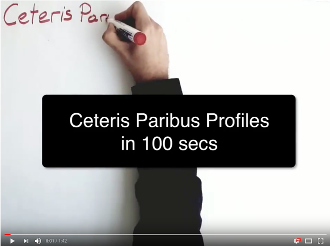Find more about Ceteris Paribus Profiles in this Gentle introduction to DALEX with examples or in the cheatsheet presented below.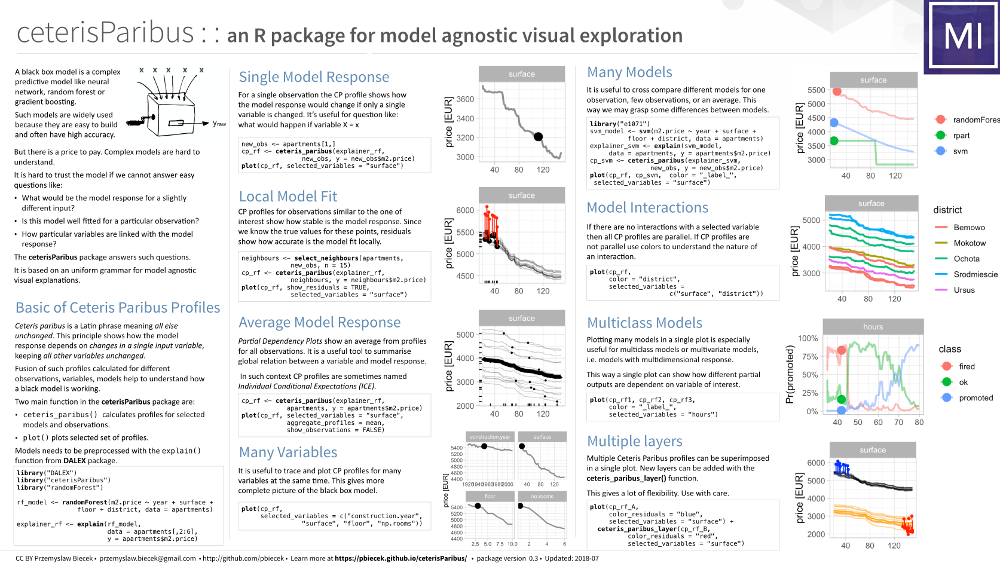Installation

install.packages("ceterisParibus")

or from GitHub:

source("https://install-github.me/pbiecek/ceterisParibus")

Simple Ceteris Paribus Plot

The plot below shows Ceteris Paribus plots for an apartment data for a large apartment (130 m2) on 3-rd floor. On can read what would be the model prediction for smaller apartment (largest change) or one that is located higher.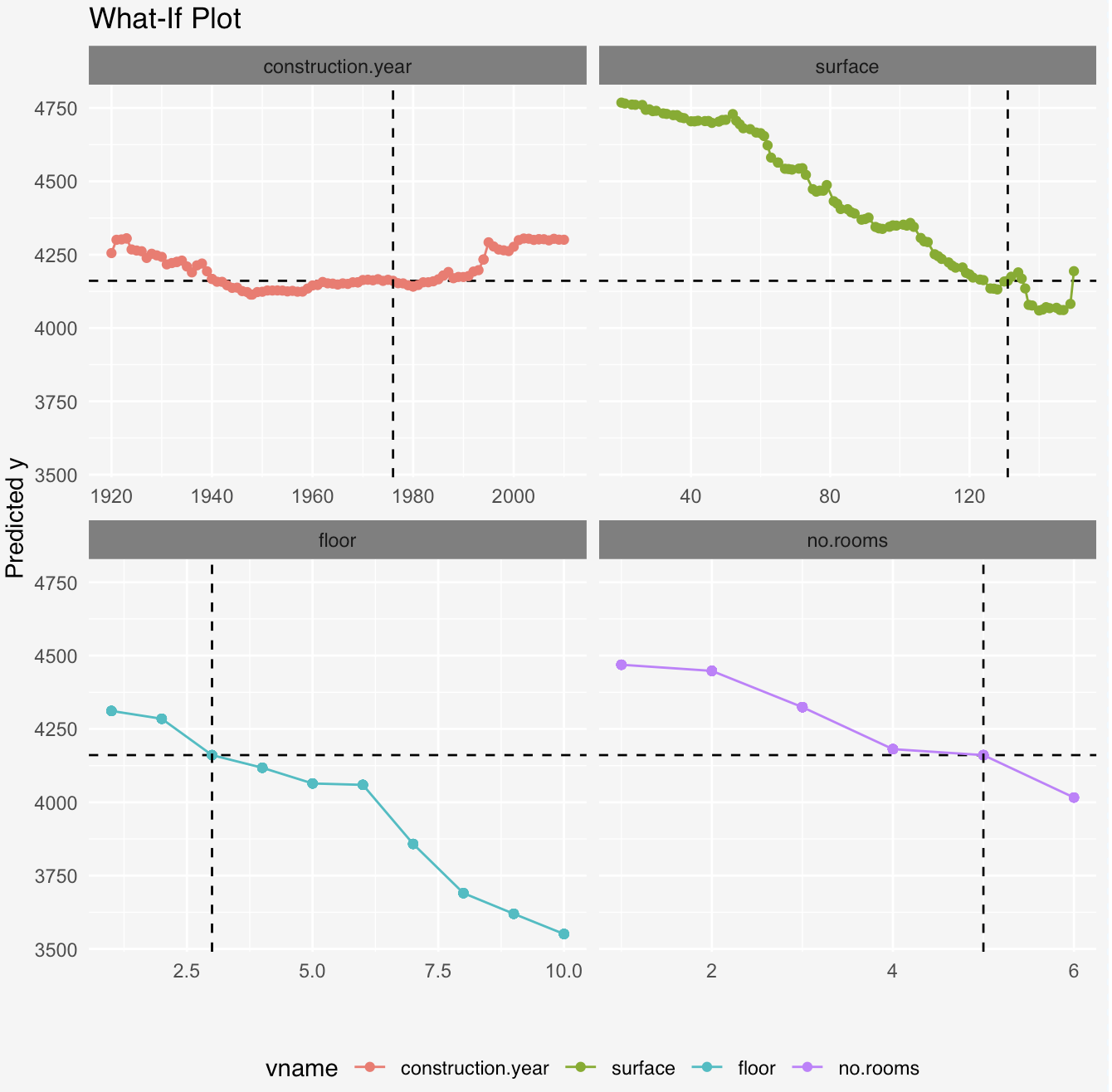Uniform Ceteris Paribus Plot

Since different variables may have different scales, we recommend to transform variables into quantiles. Then it is much easier to see how a particular variable looks in relation to others.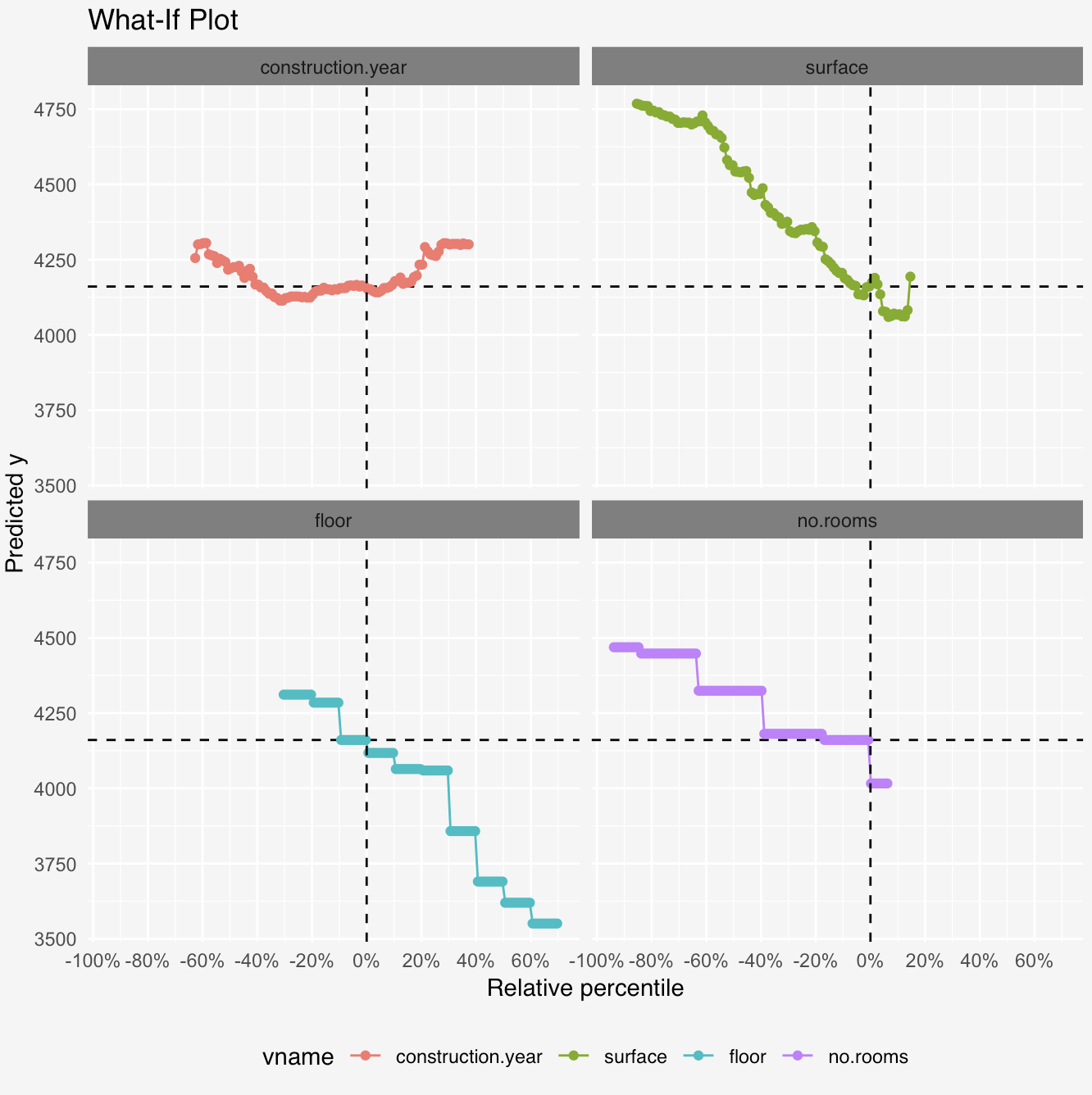Having all variables in a single quantile scale we may plot them together in a single plot.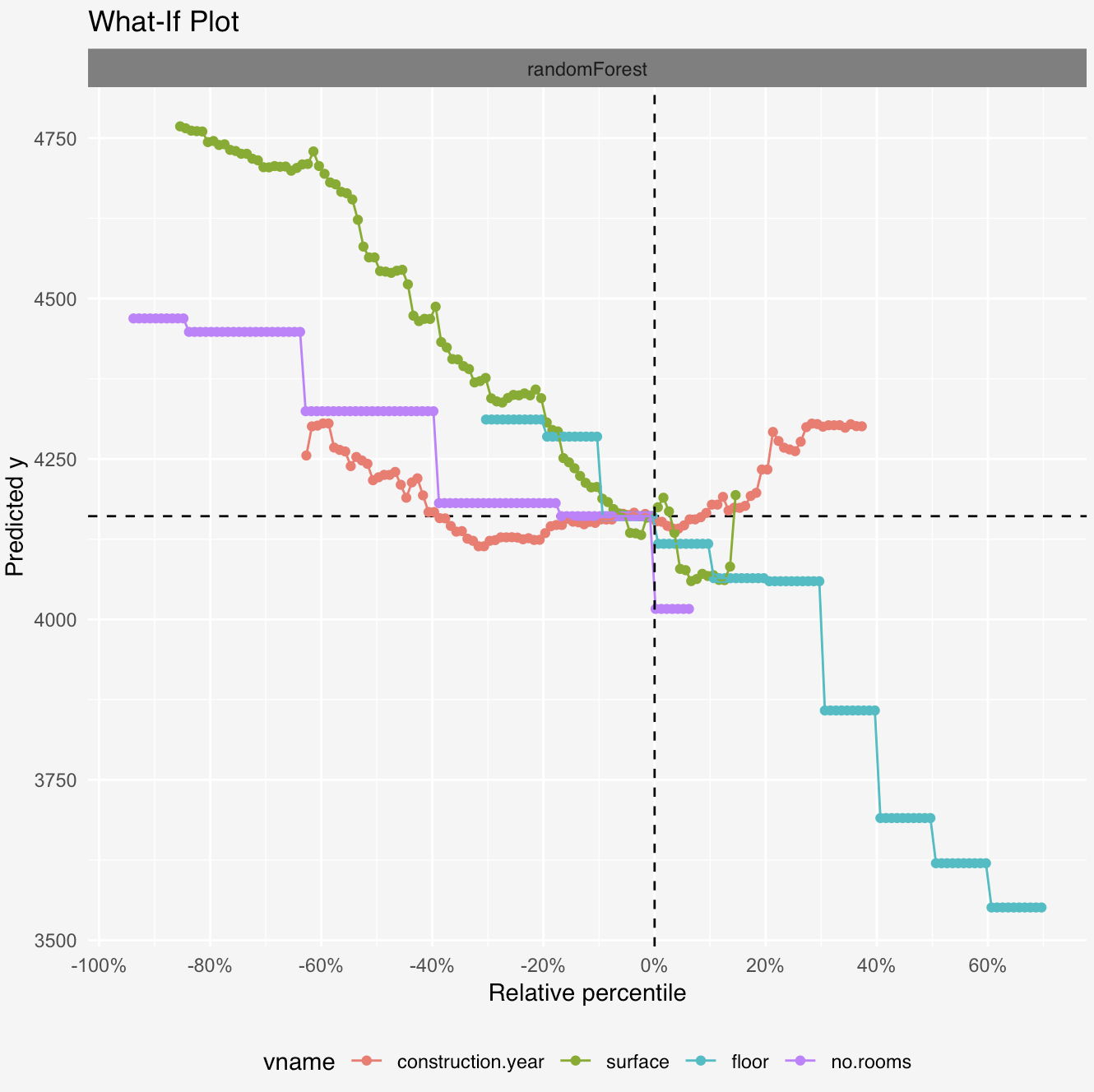Multimodel Ceteris Paribus Plot

We may compare responses for few models in a single plot.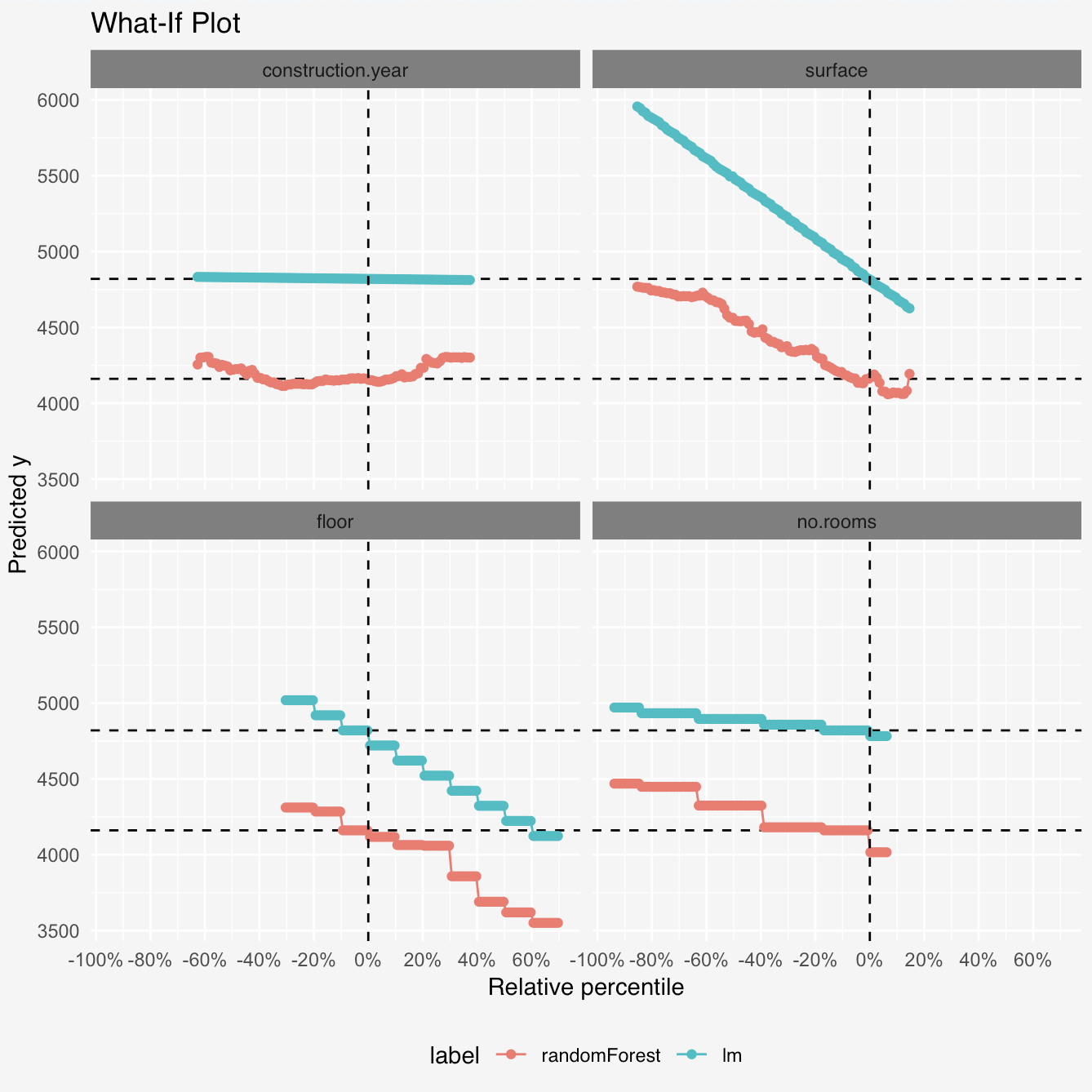Some equations

More formally, a Ceteris Paribus plot for variable V and observations O is a set of points (X, Y) where

$$Y(X) := f(O_1, …, O_{V-1}, O_{V} + X, O_{V+1}, …, O_{p})$$